Do you like the article?
Share it with others -
post a link to it!
Use new possibilities of MetaTrader 5

# Drawing Channels - Inside and Outside View

16 February 2011, 16:54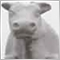8
8 651

### Introduction

I guess it won't be an exaggeration, if I say the channels are the most popular tool for the analysis of market and making trade decisions after the moving averages. In the first article of the series, which is devoted to the channels, we are going to discuss the mathematical basis and the theoretical implementation of an indicator that draws a channel set by three extremums on the screen of the client terminal.

At the first sight, drawing of a channel seems to be an easy task, since it is based on the equation of a straight line, which is taught in primary school. However, its practical implementation in the client terminal involves a lot of questions that cannot be answered straight forward.

How to organize setting of extremum and tracking of their changes in a best way? What to do and how to draw a channel, if its middle part lies on the missed bars? What if the left extremum of a channel is on Friday and the right one is on Monday, so the days off without bars are between them? How can we get the current values of a channel borders?

These and some other questions are answered in the first article of the series of articles about channels. Here you can also find the implementation of drawing of channels by three specified extremums using the standard classes and the object-oriented approach. We are going to implement the channel drawer in the form of an indicator.

### Setting Extremums

In fact, the position of a channel on a chart is determined by at least three extremums. If we give a definition to an extremum then we can accept this one: it is the maximal or minimal value of a function on a given range. A point where an extremum is reached is called an extremum point. Respectively, if a minimum is reached then the extremum point is called a minimum point, and if it's a maximum then it's called a maximum point.

The mathematical analysis defines another term - a local extremum (the minimum and the maximum one, respectively). In the maximum (minimum) point, the function value is greater (smaller) than the values of all adjacent points. The definition is take from Wikipedia (translated from Russian).

For the purpose of drawing channels we need local extremums. Let's show it graphically without going into the mathematical formulas. On the figure 1 located below, there are three local extremums marked with the red price levels. The rectangle points show two maximums and one minimum:Figure 1. The examples of local extremums

Not all the existing extremum are marked on the chart, there are only the most significant ones. For a candlestick or a bar chart it is convenient to use the "fractal" term for defining extremums - when several adjacent bars to the left and to the right are strictly descending or ascending (see fig.1).

Since we don't have a purpose to make an automatic channel drawer, then the position of extremums will be set as is shown in fig. 1 - by the position on the time and prices axes. The most suitable for this purpose are price labels - the special graphical objects of the MetaTrader 5 client terminal. A price label has the time and price coordinate properties, what allows to definitely identify an extremum point on a chart.

### The object for storing extremums is the class TExtremum

The first thing we are to do is to develop a container-class for storing extremums and a class for manipulating a group of extremums. Since we are going to use the standard classes included in the terminal as much as it is possible, the class TExtremum will be inherited from the standard class CObject. The description of our class is given below:

```class TExtremum : public CObject
{
private:
datetime  extr_datetime;              // data/time in an extremum point
double    extr_price;                 // price in an extremum point

protected:
virtual int  Compare(const CObject* _node, int _mode = 0) const;

public:
void      TExtremum();               // constructor
void      ~TExtremum();              // destructor
void      SetExtremum(datetime _time, double _price);  // change date/time and price in an extremum point
void      SetDateTime(datetime _time);                 // change date/time in an extremum point
void      SetPrice(double _price);  // change price in an extremum point

public:
datetime  GetDateTime() const;      // get date/time in an extremum point
double    GetPrice() const;         // get price in an extremum point

public:
virtual bool  SaveExtremum(string _dt_name, string _p_name);  // save extremum
virtual bool  LoadExtremum(string _dt_name, string _p_name);  // load extremum
virtual bool  DeleteExtremum(string _dt_name, string _p_name);// delete extremum
};```

Most of methods are trivial and are not worth of paying our attention to their implementation. The thing we should linger on is the TExtremum::Compare method. This method is declared in the CObject class and is used for sorting within a list. We have implemented in the following way:

```//---------------------------------------------------------------------
//  Comparing two extremums by time:
//---------------------------------------------------------------------
int TExtremum::Compare(const CObject* _node, int _mode = 0) const
{
datetime  temp = ((TExtremum* )_node).GetDateTime();
datetime  curr = GetDateTime();
if(curr > temp)
{
return(_mode > 0 ? 1 : -1);
}
else if(curr < temp)
{
return(_mode > 0 ? -1 : 1);
}

return(0);
}```

The parameter _mode here is intended for setting a direction of sorting. If it is greater than zero, then sorting is direct (ascending), otherwise it is reverse (descending).

In addition to it, there are two methods intended for saving/loading an extremum. Let's store our extremum in global variables. Here are those methods:

```//---------------------------------------------------------------------
//  Save extremum (date/time):
//---------------------------------------------------------------------
bool TExtremum::SaveExtremum(string _dt_name, string _p_name)
{
datetime  dt_result = GlobalVariableSet(_dt_name, (double)extr_datetime);
datetime  p_result = GlobalVariableSet(_p_name, (double) extr_price);
if(dt_result != 0 && p_result != 0)
{
return(true);
}

return(false);
}

//---------------------------------------------------------------------
//  Load extremum (date/time):
//---------------------------------------------------------------------
bool TExtremum::LoadExtremum(string _dt_name, string _p_name)
{
double  dt_temp, p_temp;
bool    result = GlobalVariableGet(_dt_name, dt_temp);
result &= GlobalVariableGet(_p_name, p_temp);
if(result != false)
{
extr_datetime = (datetime)dt_temp;
extr_price = p_temp;
return(true);
}

return(false);
}```

Two methods of reading/writing in global variables TExtremum::LoadExtremum and TExtremum::SaveExtremum return 'true' in case of successful execution.

### Manipulating the List of Extremums - the Class TExtremumList

Since we need both storing and sorting of extremums by time, then we should inherit the class TExtremumList from the standard class CList. With this inheritance we get a universal manipulator of extremums without limits on their number and type. This allows further expanding of number of channels that are drawn. For example, we can add drawing of the channel if non-linear regression by several extremums.

The description of this class is given below:

```class TExtremumList : public CList
{
private:
string              channel_prefix;     // channel name (prefix)
ENUM_TIMEFRAMES      chart_timeframe;    // current timeframe
string              chart_symbol;       // work symbols of the chart

protected:
string    MakeDTimeName(int _nmb);     // get name for saving/reading data/time of an extremum
string    MakePriceName(int _nmb);     // get name for saving/reading price of an extremum

public:
void      TExtremumList();             // конструктор
void     ~TExtremumList();             // деструктор
void     SetChannelParams(string _pref, string _symbol = NULL, ENUM_TIMEFRAMES _curr_tf = PERIOD_CURRENT);
void     AddExtremum(datetime _time, double  _price);
void     DeleteAllExtremum();
void     SaveExtremumList();
void     LoadExtremumList();
int      FindExtremum(datetime _dt);  // search extremum by specified time

public:
datetime GetDateTime(int _index);
double   GetPrice(int _index);
};```

Main method of class is TExtremumList::AddExtremum. It is intended for adding a new extremum to the list. The sorting of extremums in the list by extremum point time is performed after adding. The code of this method is given below:

```void TExtremumList::AddExtremum(datetime _time, double  _price)
{
//  Create extremum:
TExtremum*    extr = new TExtremum();
extr.SetExtremum(_time, _price);

//  Add it in the list:
Add(extr);

//  Sort:
Sort(1);
}```

The following methods of the base class are used here: CList::Add - for adding a new element to the list, and CList::Sort - for sorting elements in the list. The method TExtremum::Compare is used in CList::Sort.

Let's take a look at the method of searching an extremum with the given time in list TExtremumList::FindExtremum. The code of the method is given below:

```int TExtremumList::FindExtremum(datetime _dt)
{
int           k = 0;
TExtremum*    extr = (TExtremum*)(GetFirstNode());
while(extr != NULL)
{
if(extr.GetDateTime() == _dt)
{
return(k);
}
extr = (TExtremum*)(GetNextNode());
}
return(-1);                     // extremum not found
}```

The following methods of the base class are used here: CList::GetFirstNode - for getting the first element of the list (if the list is empty, it returns a zero pointer), and CList::GetNextNode - for getting next element of the list (if there is no next element and the list is over, a zero pointer is returned).

Note:

There is a pointer to a current element in the internal data of the class list CList. This pointer is changed when calling methods of moving in the list (CList::GetFirstNode, CList::GetNextNode, CList::GetPrevNode, etc.). If none of such methods has been called before, the pinter to a current element points to the first one.

In case an extremum with the given time is successfully found, the method TExtremumList::FindExtremum index of the found element. If there is no such element, it returns -1.

The methods TExtremum::MakeDTimeName and TExtremum::MakePriceName are auxiliary. They are intended for getting names of global variables that are used when saving and reading extremums. These methods have the following implementation:

```string TExtremumList::MakeDTimeName(int _nmb)
{
string    name;
StringConcatenate(name, channel_prefix, "_", channel_symbol, "_", channel_timeframe, "_DTime_Extr", _nmb);
return(name);
}

string TExtremumList::MakePriceName( int _nmb )
{
string    name;
StringConcatenate(name, channel_prefix, "_", channel_symbol, "_", channel_timeframe, "_Price_DTime_Extr", _nmb);
return(name);
}
```

An example of obtained name: "MainChannel_EURUSD_5_DTime_Extr1". Such name corresponds to a temporary extremum point of the channel MainChannel (conventional name), the symbol EURUSD, the timeframe 5M and the extremum number 1. The number for an extremum is assigned in order of ascending of its time, starting from 1. Practically, it is the index shifted on 1 in an ascending sorted list.

An example of value of three extremums saved in the terminal is show in the figure below:Figure 2. The extremums stored in the global variables

The classes described above are attached to the article in the file ExtremumClasses.mqh.

### Indicator for Setting the Extremums Manually - ExtremumHandSet

Well, we have everything required for developing the first indicator, using which we will set the position of extremums in the manual mode. The code of the indicator is attached to the article in the file ExtremumHandSet.MQ5. Let's analyze its drawing in details.

First of all, let's imagine visually what we want to see on the screen: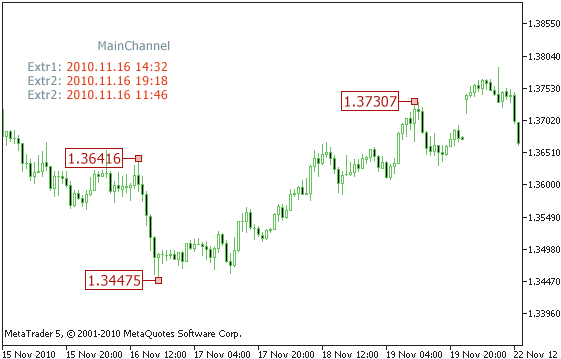Figure 3. The indicator of setting extremums

Using the left price labels we set positions of extremums on the time and price axes of the chart. The indicator must determine the position of those labels on the chart, display temporary extremum points on the screen and save them in the global variables of the clients terminal in the format described above. In addition, the indicator must track moving of the price labels on the chart and correct the loaded temporary extremum points.

The tracking of moving of the price labels on the chart will be performed once a second. This will allow the system being independent of coming of quotes and working/non working days.

Firstly, let's connect the required libraries:

```//---------------------------------------------------------------------
//  Included libraries:
//---------------------------------------------------------------------
#include  <TextDisplay.mqh>
#include  <ExtremumClasses.mqh>
```

The first library contains the classes for organization of displaying of text information on the screen (see. the article "Create your own Market Watch using the Standard Library Classes"). Using it, we are going to display the values of temporary extremum points.

Then we add the input parameters of the indicator (only the main ones are described here):

```input string  PrefixString = "MainChannel";
//---------------------------------------------------------------------
input color   ExtremumPointColor = Yellow;
//---------------------------------------------------------------------
input bool    ShowInfo = true;```

The first parameter PrefixString sets a prefix that is used for composing names of global variables when writing/reading an extremum. It also gives a possibility of using several indicator of this kind on a single chart. The only thing to do is to set different prefixes for them.

The parameter ExtremumPointColor sets a color for left price labels that determine the position of extremums. The price labels must be of a specified color. This accordance is checked in the indicator. Labels with different parameters are ignored.

The parameter ShowInfo controls displaying of the text information about the specified extremum points on the screen.

Next, let's create the objects for displaying of information and manipulating the extremums:

```TableDisplay    TitlesDisplay;    // displaying information on the screen
//---------------------------------------------------------------------
TExtremumList*  PrevExtr_List;    // list of previous extremums
TExtremumList*  CurrExtr_List;    // list of current extremums
TExtremumList*  NewExtr_List;     // list of new extremums```

These objects are initialized in the following:

```PrevExtr_List = new TExtremumList();
PrevExtr_List.SetChannelParams(PrefixString, Symbol(), Period());
PrevExtr_List.LoadExtremumList();

CurrExtr_List = PrevExtr_List;

NewExtr_List = new TExtremumList();
NewExtr_List.SetChannelParams(PrefixString, Symbol(), Period());```

In the list PrevExtr_List we load extremums from the global variables using the method TExtremumList::LoadExtremumList. This list will store the extremums for comparing them with new ones, which will be read from a chart when dragging the price labels on the screen.

The list CurrExtr_List is used as a current one, it stores current extremums. Since in the beginning we have only the extremums read from the global variables, they are taken as the actual ones.

In the list NewExtr_List we are going to write new extremums found on the chart.

Let's take a look at main functions that are used in the indicator. The first function FindExtremumPoints is used for reading and checking the parameters of price labels that determine the position of extremums:

```bool FindExtremumPoints(long _chart_id)
{
string  name;

//  1. Search for the total number of objects with specified parameters and write them to the list:
int total_objects = ObjectsTotal(_chart_id, -1, OBJ_ARROW_LEFT_PRICE);
if(total_objects <= 0)
{
return(false);
}

NewExtr_List.Clear();
for(int i = 0; i < total_objects; i++)
{
name = ObjectName(_chart_id, i, -1, OBJ_ARROW_LEFT_PRICE);

if( IsGraphicObjectGood(_chart_id, name, OBJ_ARROW_LEFT_PRICE, ExtremumPointColor) == true)
{
NewExtr_List.AddExtremum(ObjectGetInteger( _chart_id, name, OBJPROP_TIME),
ObjectGetDouble(_chart_id, name, OBJPROP_PRICE));
}
}

//  2. If three extremums are found, we can try to draw a channel:
if(NewExtr_List.Total() == 3)
{

//  Save the list of new extremums:
NewExtr_List.SaveExtremumList();
return(true);
}

NewExtr_List.Clear();
return(false);
}```

First of all, the list NewExtr_List is cleared by calling the method TExtremumList::Clear, and then all the found extremum points, which have the specified parameters, are added to it. If the number of found points is three, then the list is saved in the global variables and the function returns 'true'.

The other function CheakExtremumMoving tracks moving of the extremum points on the chart. If at least on point is moved along the time axis of the chart, this function returns 'true'.

Its code is given below:

```//---------------------------------------------------------------------
//  Check whether extremums have been moved on the screen:
//---------------------------------------------------------------------
bool CheakExtremumMoving()
{
if(FindExtremumLines(0) == true)
{
int  count = NewExtr_List.Total();
int  index;
for(int i = 0; i < count; i++)
{
index = CurrExtr_List.FindExtremum(NewExtr_List.GetDateTime(i));

//  If a new extremum is found:
if(index == -1)
{
PrevExtr_List = CurrExtr_List;
CurrExtr_List = NewExtr_List;
return(true);
}
}
CurrExtr_List = PrevExtr_List;
}

return(false);
}```

We've considered the way of setting extremum points in the manual mode. We have the ready indicator that allows controlling this process and writing the points to the global variables. The full code of the indicator is in the attached file ExtremumHandSet.mq5. Now we can move to the main part - drawing a channel.

### Drawing a Channel - Some Theory

A linear channel consists of two parallel lines that go strictly through extremum points. In addition, one line must go through two points, and the other must go through the one that is left being parallel to the first line. It can be shown in a simple picture:Figure 4. Drawing a channel using three extremum points

As we know from geometry, only one straight line can be drawn through two points. This line has the red color in the fig. 4. It goes thought two points that have the following coordinates - (T1, P1) and (T2, P2); the points are marked with the letters A and B. The equation of this line is:

(1)   P(t) = P1 + (t - T1)*(P2 - P1) / (T2 - T1); P(t) here is the price calculated at the time 't'.

Through the point C (the third extremum), we should draw another straight line parallel to the first one. This line has the green color in the fig. 3. Since the T1 and T2 points are the same for both lines, we should find the values of P1' and P2' (see. fig. 4).

Before moving forward, we need to make an important remark. The terminal chart doesn't display time "holes". For example, the days off, when quotes don't come to the terminal, should be displayed as breaks of prices. And it is bad that they are not. What is the point in looking at an empty chart? However, if we use absolute time in the equation above, we will get a wrong channel.

Fortunately, the situation is not hopeless. If we change the absolute time to the relative number of a bar, then we'll be able to use those coordinates for drawing a channel, because the enumeration of bars cannot have breaks (practically, it is an index in a price array).

If we go further and assume that the point A in the fig. 4 always lies on a zero coordinate (zero bar) of the time axis, then our equation will become even easier. So, T1=0, T3=B3,Т2=В2. В3 and В2 here are the numbers of a bar relatively to the point Т1 (i.e. the zero point). It is clear that this assumption doesn't lead to inclining of the line. Then we get the following equation of a straight line that goes through the points A and B:

(2)   P(n) = P1 + n * (P2-P1) / B2, where P(n) is the price calculated for a bar that has the 'n' number.

So, we know the values P1, P2, P3 and B2, B3. Now we need to find the P1' and P2' values. Combining two equations and solving them, we get the following formulas, using which we can find the unknown values:

(3)   P1' = P3 - B3 * (P2 - P1) / B2

(4)   P2' = P2 - P1 + P1'

As we find the value P1' and substitute it to the (4) formula, we will get the P2' value. Now we have the theoretical basis for drawing a channel. Let's start implementing it.

### Drawing Channel Borders - the Class TChannelBorderObject

This class is derived from the standard class CChartObjectTrend. Its purpose is storing all the parameters connected with the borders of a channel as well as drawing/deleting the border lines and controlling the graphical parameters of these lines.

The description of this class is given below:

```class TChannelBorderObject : public CChartObjectTrend
{
//  General properties of a border:
private:
bool             is_created;       // whether the graphical object is created on the screen
long             chart_id;         // identifier of the chart window
int              window;           // identifier of the subwindow

//  Parameters of a border line:
private:
string           border_name;      // name of the border line
color            border_color;     // color of the border line
int              border_width;     // thickness of the border line
ENUM_LINE_STYLE   border_style;     // style of the border line

//  Coordinates of a border:
private:
datetime         point_left;       // time of the left point (T1)
datetime         point_right;      // time of the right point (T2)
double           price_left;       // price of the left point (P1)
double           price_right;      // price of the right point (P2)

public:
void     TChannelBorderObject();  // constructor
void    ~TChannelBorderObject();  // destructor
bool     IsCreated();             // check whether the line is created

//  Creating/deleting a line:
public:
bool     CreateBorder(long _chart_id, int _window, string _name, datetime _t_left, datetime _t_right,
double _p_left, double _p_right, color _color, int _width, ENUM_LINE_STYLE _style);
bool     CreateBorder(long _chart_id, int _window, string _name, datetime _t_left, datetime _t_right,
double _p_left, double _p_right);
bool     CreateBorder(datetime _t_left, datetime _t_right, double _p_left, double _p_right);
bool     RemoveBorder();          // delete line from the chart

//  Setting parameters of the line:
public:
void     SetCommonParams(long _chart_id, int _window, string _name);
bool     SetBorderParams(color _color, int _width, ENUM_LINE_STYLE _style);
bool     SetBorderColor(color _color);
bool     SetBorderWidth(int _width);
bool     SetBorderStyle(ENUM_LINE_STYLE _style);

//  Getting values on the line:
double   GetPrice(datetime _dt); // get price value in the specified position of the border line
};```

This class doesn't need some special comments.

Let's pay our attention only to the method of getting the border price in a specified point:

```//---------------------------------------------------------------------
//  Get price value in the specified position of the border line:
//---------------------------------------------------------------------
double TChannelBorderObject::GetPrice(datetime _dt)
{
//  If the graphical object is created:
if(is_created == true)
{
return(ObjectGetValueByTime( chart_id, border_name, _dt));
}
return(0.0);
}```

The function of the terminal ObjectGetValueByTime is used here; it returns the price value for a specified time. It is convenient to use the terminal possibilities instead of calculating the value using a mathematical formula.

### Drawing a Channel - the Class TSlideChannelObject

This class is derived from the standard class CList. Its purpose is following:

• storing objects of the class TChannelBorderObject and performing different actions with them;
• calculating points for drawing required lines that compose a channel;
• storing and modifying the parameters of a channel;
• getting calculated values that describe a drawn channel (its height, price values on borders, etc.);

The code that describes this class is too big to be shown here completely. Those who want can see it in the file SlideChannelClasses.mqh attached to the article. Let's analyze some main parts of it.

First of all, it is getting of B2 and B3 values in the points T2 and T3 respectively (see fig.4). The following code is used:

```//  Get relative shifts in bars relatively to the extremum points:
total_bars = Bars(symbol, time_frame);     // total number of bars in history
if(total_bars == 0)
{
return(false);                           // channel cannot be drawn
}
double  B2 = Bars(symbol, time_frame, point_left, point_right);
double  B3 = Bars(symbol, time_frame, point_left, point_middle);```

To avoid a situation of calling absent bars, we use the terminal function Bars that returns the number of bars in history for a specified symbols and period. If the information is not formed yet, the function will return a zero value; it is used for checking.

If the function returns a non-zero value, then we can get the В2 and В3 values. It is done using the same function Bars but calling it in the other form. We set time limits and get the number of bars within this range. Since our left border is the same, we get the shift in bars for the points Т2 and Т3. The shift for the point Т1 is always equal to zero.

Now we can calculate all the point of the channel lines. There can be maximum nine of them, since our channel will display (in addition to the upper and lower borders) the middle line and the lines of percentage zones around the borders and the middle line.

Let's analyze the main part of the calculation. The entire calculation is in the method TSlideChannelObject::CalcChannel.

```//  Coefficient of the line inclination:
koeff_A = (price_right - price_left) / B2;

//  Price value on the AB line in the point T3:
double  P3_AB = price_left + B3 * koeff_A;

// Determine the channel type - 2MAX_1MIN или 1MAX_2MIN:
if(P3_AB > price_middle)              // 2MAX_1MIN
{
channel_type = CHANNEL_2MAX_1MIN;

left_prices[BORDER_UP_INDEX] = price_left;
right_prices[BORDER_UP_INDEX] = price_right;

left_prices[BORDER_DN_INDEX] = price_middle - B3 * koeff_A;
right_prices[BORDER_DN_INDEX] = left_prices[BORDER_DN_INDEX] + (price_right - price_left);
}
else if(P3_AB < price_middle)         // 1MAX_2MIN
{
channel_type = CHANNEL_1MAX_2MIN;

left_prices[BORDER_DN_INDEX] = price_left;
right_prices[BORDER_DN_INDEX] = price_right;

left_prices[BORDER_UP_INDEX] = price_middle - B3 * koeff_A;
right_prices[BORDER_UP_INDEX] = left_prices[BORDER_UP_INDEX] + (price_right - price_left);
}
else
{
return( false );                      // channel cannot be drawn (all extremums are on the same line)
}```

left_prices and right_prices here are the arrays that store the price coordinates of the nine lines of the channel. The time coordinate of all the lines of the channel are already known.

At first, determine the coefficient of the line inclination (see the formula (2)) koeff_A. Then we calculate the price value of the AB line in the point T3 (see fig. 4). It is done to determine, which type of the channel is specified for drawing - by two maximums and one minimum or by two minimums and one maximum. We check, which point is higher on the price axis - the point C or the point that has the (P3', T3) coordinates. Depending on their position we determine, whether the channel has the first or the second type.

As soon as the coordinates of two main lines of the channel (upper and lower) are determined, calculation of coordinates of the other seven lines is not a hard thing to do. For example, we calculate the coordinates of the middle line using the coordinates of the upper and lower borders of the channel in the following way:

```  left_prices[BORDER_MD_INDEX] = (left_prices[BORDER_DN_INDEX] + left_prices[BORDER_UP_INDEX ]) / 2.0;
right_prices[BORDER_MD_INDEX] = (right_prices[BORDER_DN_INDEX] + right_prices[BORDER_UP_INDEX]) / 2.0;```

Just take the average value from the upper and lower borders of the channel.

### Indicator for Drawing a Channel by Specified Extremums - SlideChannel

Well, we already have the class for drawing a channel. Now let's write an indicator that will read the parameters of extremums from the global variables and draw a channel on a chart. It will look as following: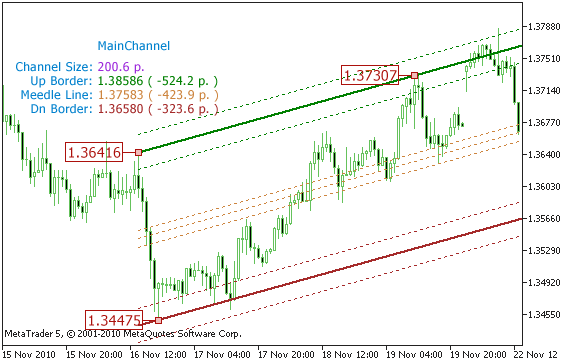Figure 5. The example of a channel drawn using extremums

The information about the drawn channel is also displayed here - its width, distance in points from the current price to the channel borders and the middle line.

Let's connect the required libraries:

```#include  <TextDisplay.mqh>
#include  <SlideChannelClasses.mqh>```

The first library contains the classes for organization of displaying of text information on the screen (see. the article "Create your own Market Watch using the Standard Library Classes"). Using it, we are going to display the values of temporary extremum points.

Then add input parameters of the indicator (only the main ones are described here):

```input string          PrefixString = "MainChannel";
//---------------------------------------------------------------------
input ENUM_TIMEFRAMES  ExtremumTimeFrame = PERIOD_CURRENT;
//---------------------------------------------------------------------
input bool            ShowInfo = true;```

The first parameter PrefixString, just the same as in the ExtremumHandSet indicator, sets a prefix that is used for composing name of the global variables when reading extremums. It also gives a possibility of using several indicator of this kind on a single chart. The only thing to do is to set different prefixes for them.

The ExtremumTimeFrame parameter sets a timeframe, which will be used for reading the extremum points from the global variables. It's a very useful parameter. It allows drawing synchronous channels on different timeframes. For example, if you set the extremums from H1, you can draw the same channel on the M5 timeframe. To do it, just add our indicator for drawing channels to the M5 chart; and it will synchronously display all the changes.

The parameter ShowInfo controls displaying of the text information about the parameters of the channel on the screen.

Next, create objects for displaying the information and drawing the channel:

```TableDisplay         ChannalDisplay;  // displaying of general information about a channel on the screen
TableDisplay         BordersDisplay;  // displaying information about the borders of a channel on the screen
//---------------------------------------------------------------------
TSlideChannelObject  Channel;         // drawing of a channel```

The object for drawing a channel is initialized in the following way:

```  Channel.CreateChannel(PrefixString, 0, 0, Symbol(), period_current, curr_left_point, curr_middle_point,
curr_right_point, curr_left_price, curr_middle_price, curr_right_price);
Channel.SetBorderWidth(BorderWidth );
Channel.SetmiddleWidth(middleLineWidth);
Channel.SetUpBorderColor(UpBorderColor);
Channel.SetDnBorderColor(DnBorderColor);
Channel.SetmiddleColor(middleLineColor );
Channel.ShowBorderZone(ShowBorderPercentageLines);
Channel.BorderZonePercentage( PercentageZoneSize);
Channel.Showmiddle(ShowmiddleLine);
Channel.ShowmiddleZone( ShowmiddlePercentageLines);
Channel.middleZonePercentage(PercentagemiddleZoneSize);
```

Here at first, we create a channel by calling the method TSlideChannelObject::CreateChannel, and then we set the required parameters of the channel line. The sequence of setting doesn't matter, you can make it vice versa - set the parameters and then create the channel.

The parameter period_current is the period that is used when reading extremums from the global variables. It may be different from the period of a current chart.

Let's take a look at main functions that are used in the indicator. The first function GetExtremums is used for reading the position of extremums and refreshing the channel according to the obtained values:

```void GetExtremums()
{
double  temp;
string  name;

StringConcatenate(name, PrefixString, "_", Symbol(), "_", period_current, "_DTime_Extr1");
if( GlobalVariableGet(name, temp) != false)
{
curr_left_point = (datetime)temp;
}

StringConcatenate(name, PrefixString, "_", Symbol(), "_", period_current, "_DTime_Extr2");
if( GlobalVariableGet(name, temp) != false)
{
curr_middle_point = (datetime)temp;
}

StringConcatenate(name, PrefixString, "_", Symbol(), "_", period_current, "_DTime_Extr3");
if( GlobalVariableGet(name, temp) != false)
{
curr_right_point = (datetime)temp;
}

StringConcatenate(name, PrefixString, "_", Symbol(), "_", period_current, "_Price_Extr1");
if( GlobalVariableGet(name, temp) != false)
{
curr_left_price = temp;
}

StringConcatenate(name, PrefixString, "_", Symbol( ), "_", period_current, "_Price_Extr2");
if( GlobalVariableGet(name, temp) != false )
{
curr_middle_price = temp;
}

StringConcatenate(name, PrefixString, "_", Symbol(), "_", period_current, "_Price_Extr3");
if( GlobalVariableGet(name, temp) != false)
{
curr_right_price = temp;
}

//  Update the position of channel:
Channel.SetExtremums(curr_left_point, curr_middle_point, curr_right_point,
curr_left_price, curr_middle_price, curr_right_price);
}```

For refreshing the channel on the screen, we use the method TSlideChannelObject::SetExtremums. This method recalculates the coordinates of the channel lines and redraws the channel on the screen.

An example of drawing a channel on different timeframes is shown in the video below:

The sequence of starting indicators doesn't really matter, but it is logical to start the ExtremumHandSet indicator at first, then add three left price labels of the yellow color (the color of labels is set in the indicator parameters, the yellow color is set on default), and the start the SlideChannel indicator that draws the channel by the specified extremums.

For a channel to be drawn synchronously with the extremums of the first chart, you should set the timeframe in the ExtremumTimeFrame parameter of the SlideChannel indicator the same as the one of the chart where the extremums are set.

This is the result of separating the function of setting the extremum points of the channel from the function of its drawing on the terminal screen.

### Conclusion

We've considered the full cycle - from setting position of a channel on the screen to its drawing. Everything appeared to be not so complicated, especially when using the standard classes and OOP.

But there is a questing: how should we use the channels for working at the market. Firstly, they are necessary for technical analysis of a current state of a financial instrument. And secondly, after the analysis, they are necessary for making decisions. The channels can help greatly in it.

It is possible to develop an semi-automatic Expert Advisor that will analyze the borders of a channel for opening or closing a position. It can work both with breaking through a border or with rolling back from it. This will be the subject of the next article - The Methods of Working with a Channel - Roll Back and Break Through.

Translated from Russian by MetaQuotes Software Corp.
Original article: https://www.mql5.com/ru/articles/200

Attached files |
extremumclasses.mqh (12.58 KB)
extremumhandset.mq5 (11.89 KB)
slidechannel.mq5 (13.7 KB)
textdisplay.mqh (15.21 KB)

#### Other articles by this author

Last comments | Go to discussion (8)| 21 Feb 2011 at 21:58
Lugner:

"Cheak" in function name should read "Check" .. just typo in the function name, it does not cause error because you call "CheakExtremumMoving"

razoff; these types of errors are all over code if you look hard enough -- simple fix will be to Find and Replace on all "CheakExtremum" to "CheckExtremum" - or obviously for other errors of this kind you find. You can Find in Files for instances and references to incorrect spellings then replace in each file; that way when integrating code from the community you can decide what to call your objects and methods.

Yes, it's my mistake :)| 27 Feb 2011 at 18:25

hi, i applied the indicator but in the top left corner it only shows 0.00000 ( 0.0 p. ), this value is constant for the upborder, middle line and dn border. what can i do to fix this problem?

thnxs| 28 Feb 2011 at 12:52
deuce:

hi, i applied the indicator but in the top left corner it only shows 0.00000 ( 0.0 p. ), this value is constant for the upborder, middle line and dn border. what can i do to fix this problem?

thnxs

Hi,

the channal system consist of two parts (indicators):

-ExtremumHandSet - set it first on chart and setup 3 extremums (3 different points)

-SlideChannal - it draw the channal

You can see on video all steps.

WBR| 18 Aug 2011 at 13:22

get lot of errors while compiling  SlideChannel.mq5 "1.01" on b489 i386 system

'BORDER_UP_INDEX' - integer expression expected    SlideChannelClasses.mqh    706    21
....| 25 Jul 2012 at 14:45

beautiful work!!!! But when I attached Extremumhandset on chart, it doesn't give me the extremes. So I don't even have to be slidechannel. Can you help me please?

Thanks a lot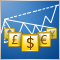The Implementation of a Multi-currency Mode in MetaTrader 5
For a long time multi-currency analysis and multi-currency trading has been of interest to people. The opportunity to implement a full fledged multi-currency regime became possible only with the public release of MetaTrader 5 and the MQL5 programming language. In this article we propose a way to analyze and process all incoming ticks for several symbols. As an illustration, let's consider a multi-currency RSI indicator of the USDx dollar index.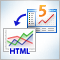Charts and diagrams in HTML
Today it is difficult to find a computer that does not have an installed web-browser. For a long time browsers have been evolving and improving. This article discusses the simple and safe way to create of charts and diagrams, based on the the information, obtained from MetaTrader 5 client terminal for displaying them in the browser.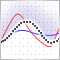Averaging Price Series for Intermediate Calculations Without Using Additional Buffers
This article is about traditional and unusual algorithms of averaging packed in simplest and single-type classes. They are intended for universal usage in almost all developments of indicators. I hope that the suggested classes will be a good alternative to 'bulky' calls of custom and technical indicators.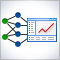Connecting NeuroSolutions Neuronets
In addition to creation of neuronets, the NeuroSolutions software suite allows exporting them as DLLs. This article describes the process of creating a neuronet, generating a DLL and connecting it to an Expert Advisor for trading in MetaTrader 5.